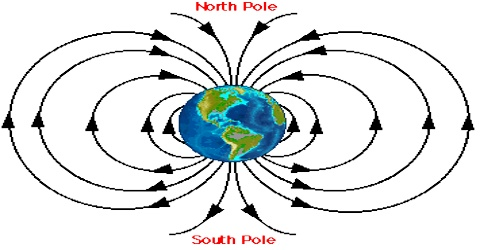Physics

# Gravitational Field of the EarthGravitational field of the earth:

A gravitational field is a model used to explain the influence that a massive body extends into the space around itself, producing a force on another massive body. The earth is considered a uniform sphere radius R. So, its total mass M is assumed to be concentrated at the centre. So, intensity of a distance from the centre of the earth (r > R) will be, E = GM/r12. Force of gravity a body of mass m is mg. So on the earth’s surface or near to it intensity, E = mg/m = g.

Hence, on the earth’s surface or near to it field intensity of the earth is equal to the acceleration due to gravity.

Intensity due to a point mass: In order to find the intensity at a distance r from a point mass of M a unit mass is placed at that point. Now according to Newton’s gravitational law, force acting on the unit mass, i.e., intensity at that point,

E = G (M x 1)/r2 = GM/r2.

A field is something that has a magnitude and a direction at every point in space. Gravity is a good example – we know there is acceleration due to gravity of about 9.8 m/s2 down at every point in the room. Another way of saying this is that the magnitude of the Earth’s gravitational field is 9.8 m/s2 down at all points in room.

Gravitational field: g = F/m

where F is the force of gravity.

We can draw a field-line pattern to reflect that, near the Earth’s surface, the field is uniform. The strength of a field is reflected by the density of field lines – a uniform field has equally-spaced field lines.